# ISC Class 12 Chemistry Question Paper Solution 2015

The ISC Class 12 Chemistry – Paper I (Theory) was conducted on 20th March 2015. The exam started at 2 PM. The paper was of 3 hours of time duration and 70 Marks. Here, we have provided the ISC Class 12 Chemistry Question Paper Solution 2015. The best way to prepare for the Chemistry exam is to solve the ISC Class 12 Chemistry Previous Year Question Papers. Also, to make the exam preparation more effective, students must assess their performance after solving past years papers. By going through the solution pdf, students can identify their mistakes and can work on them to improve their performance in the board exam. They can download the ISC Class 12 Chemistry Question Paper and Solution pdf 2015 from the link below.

Science Stream students can also access the Solved ISC Class 12 Previous Year Question Papers with Solutions for all subjects compiled at one place. They can have a look at the ISC Class 12 Chemistry Question Paper Solution 2015 below.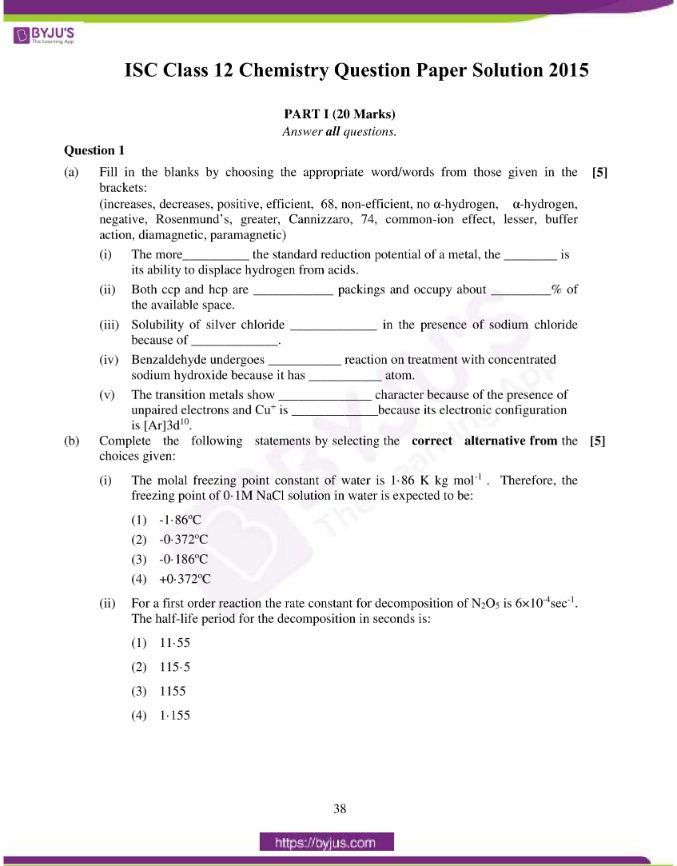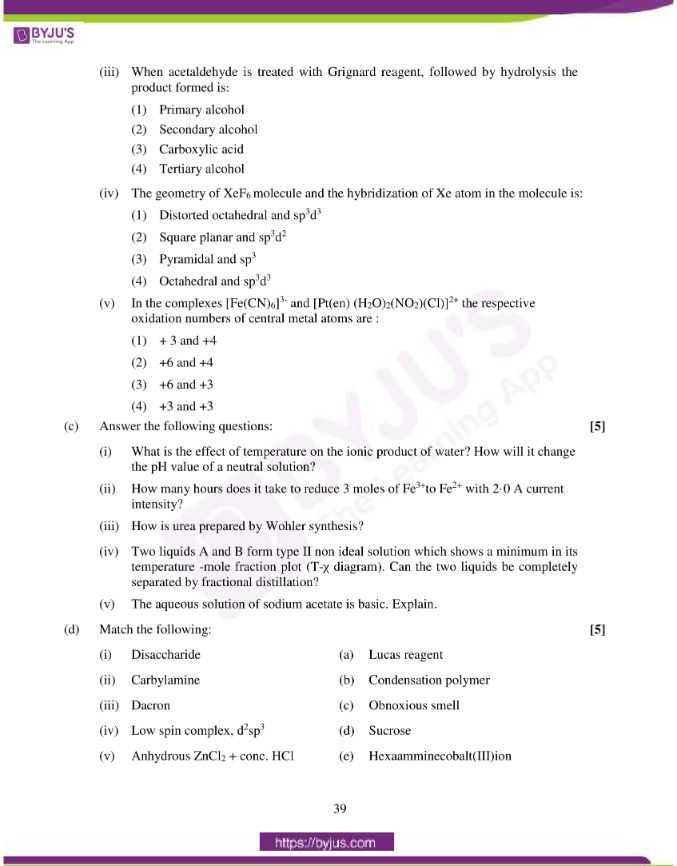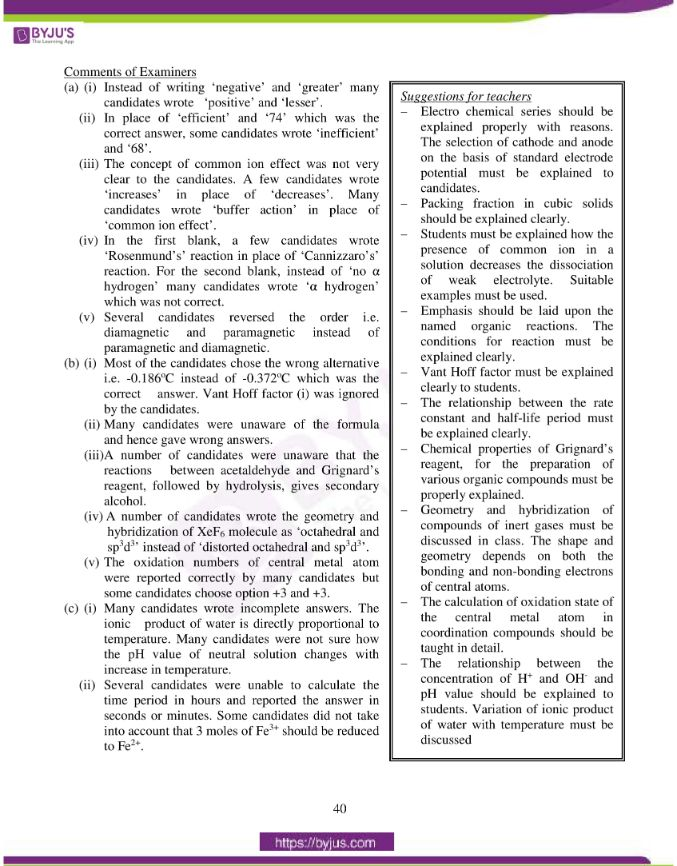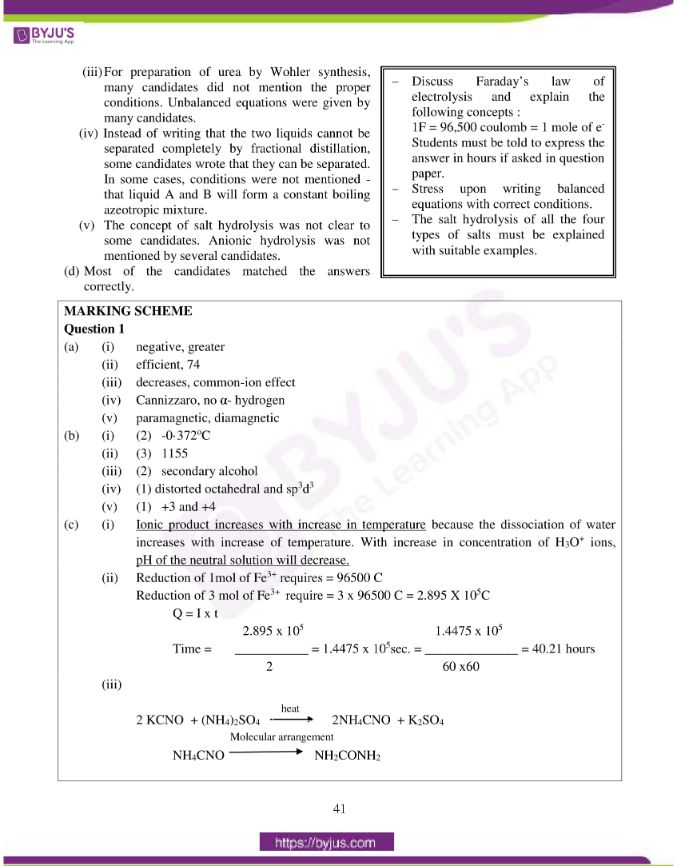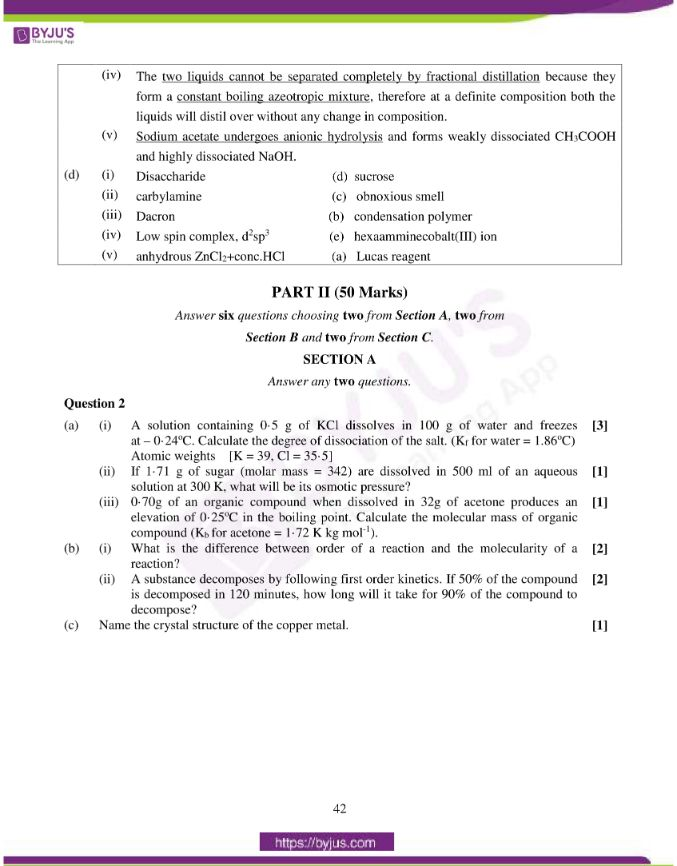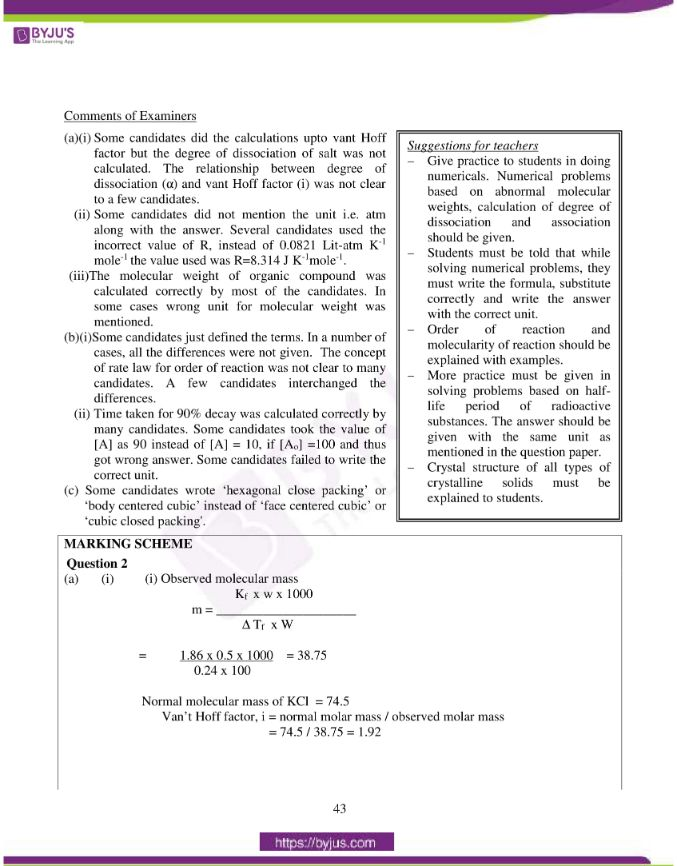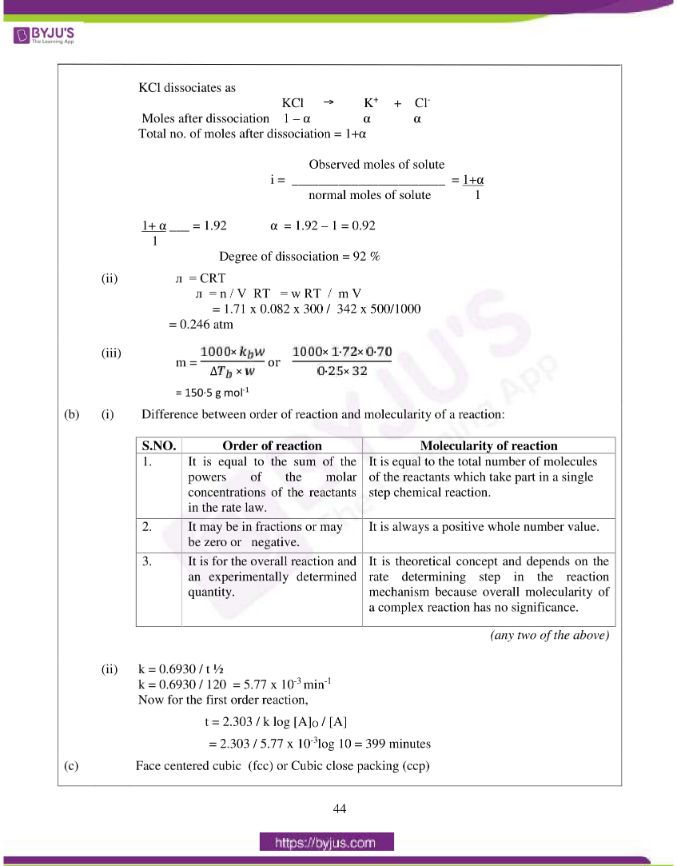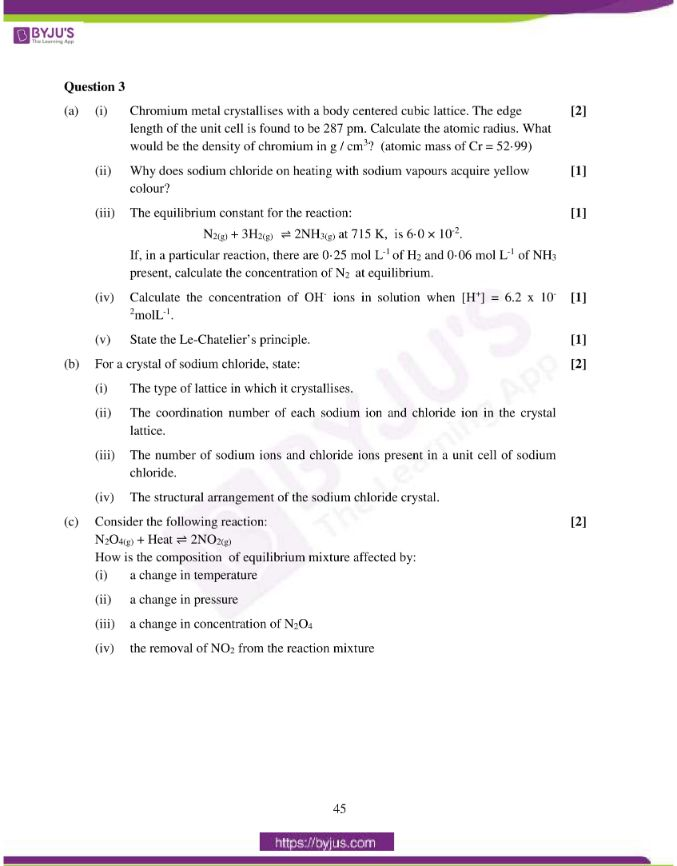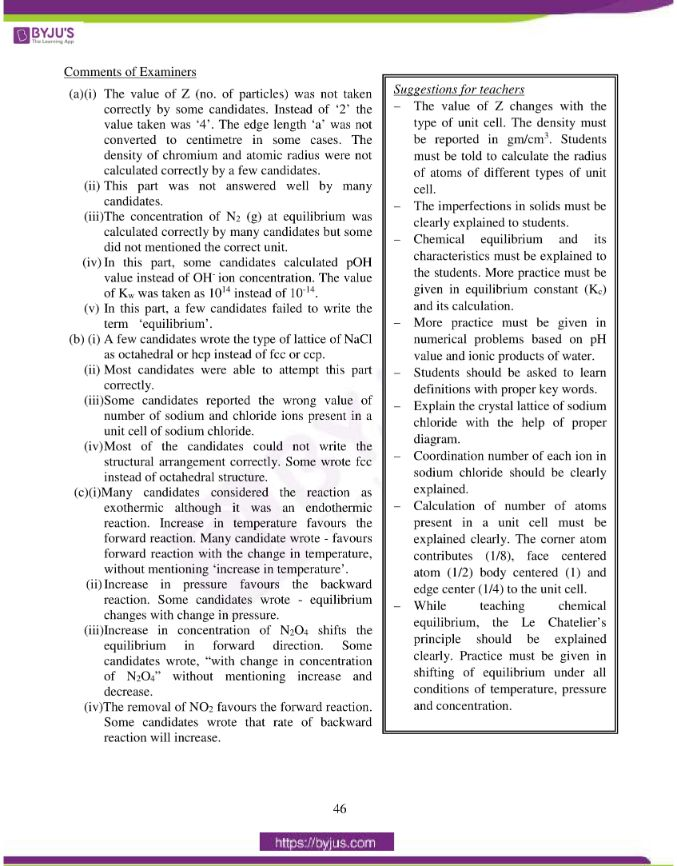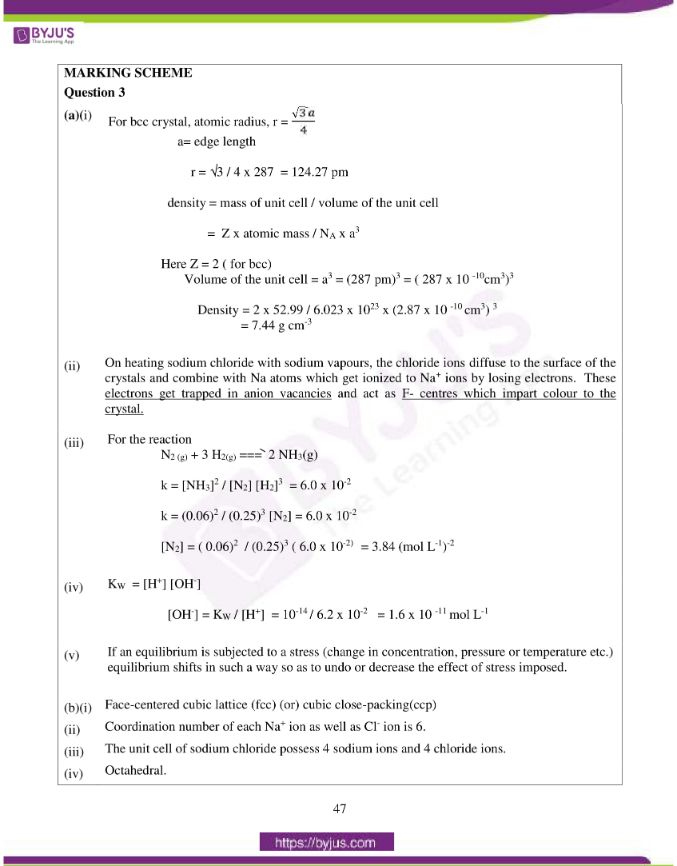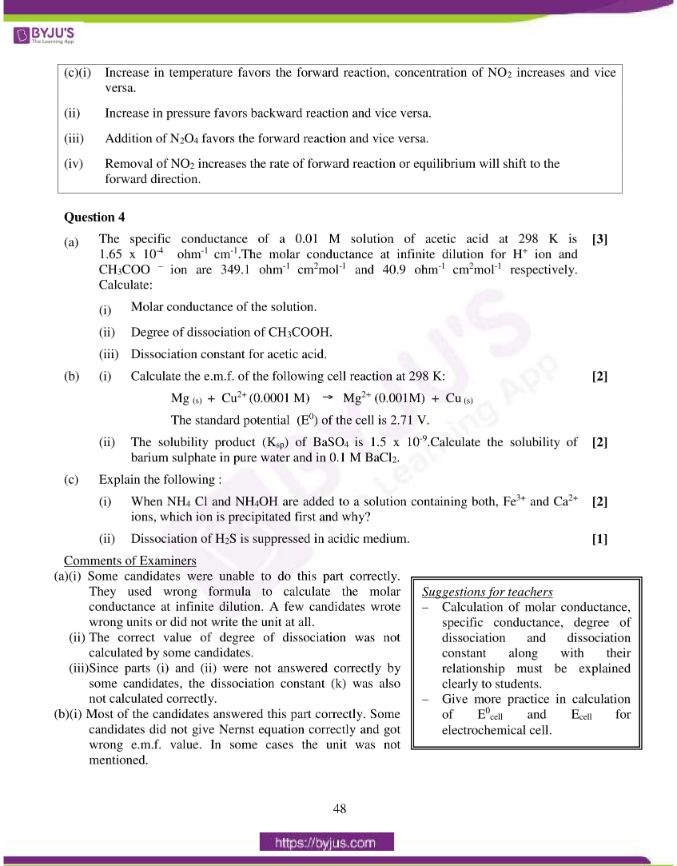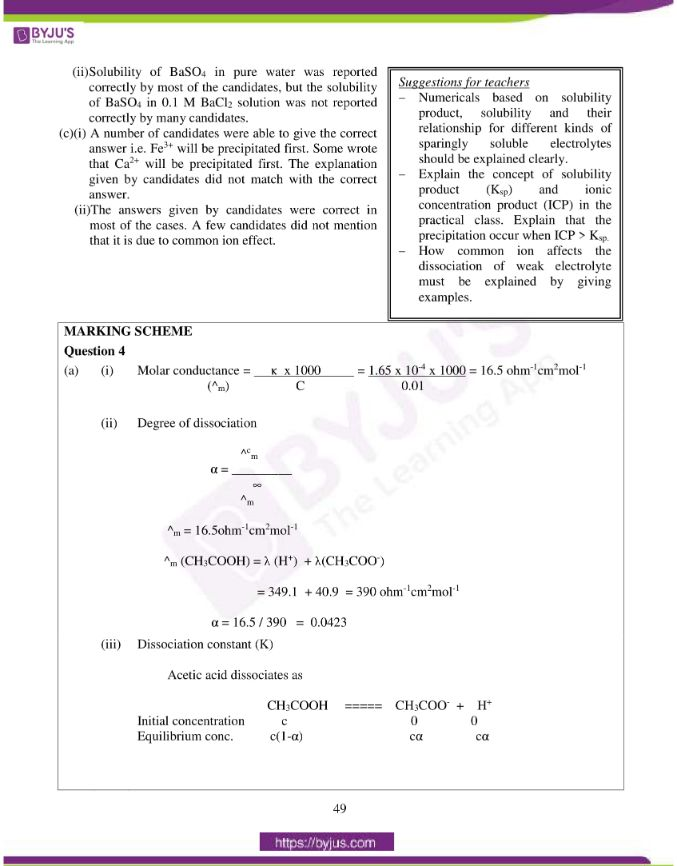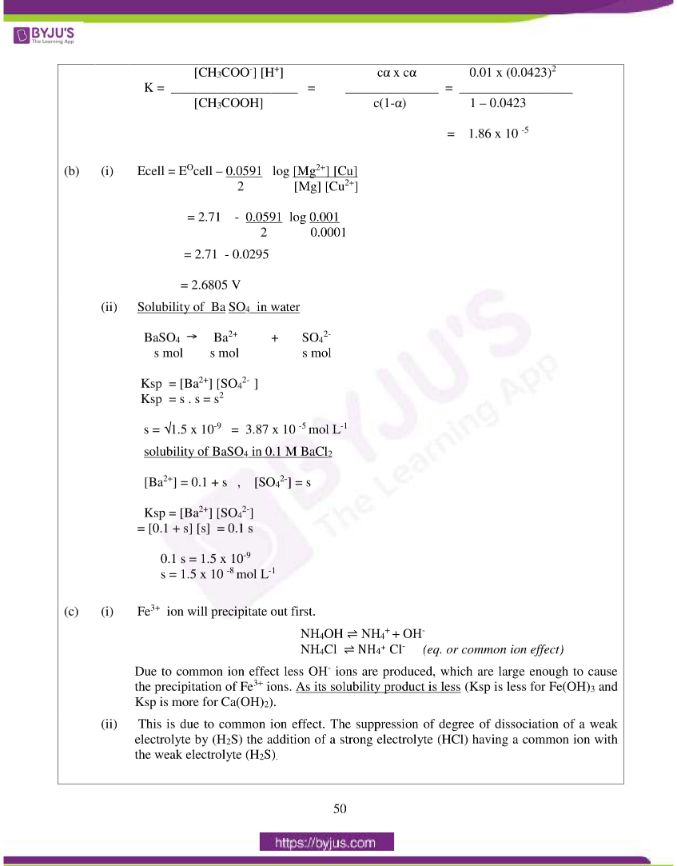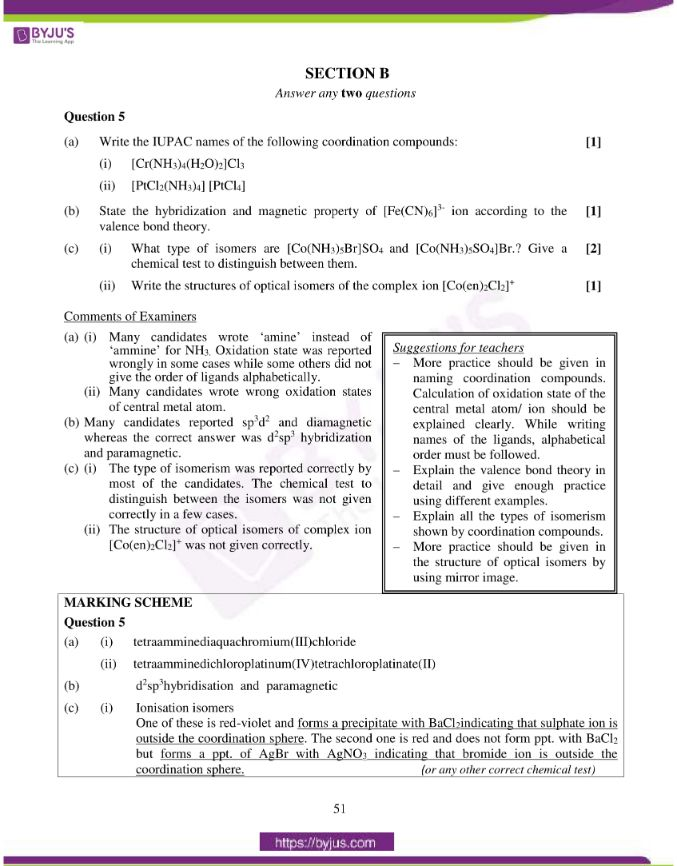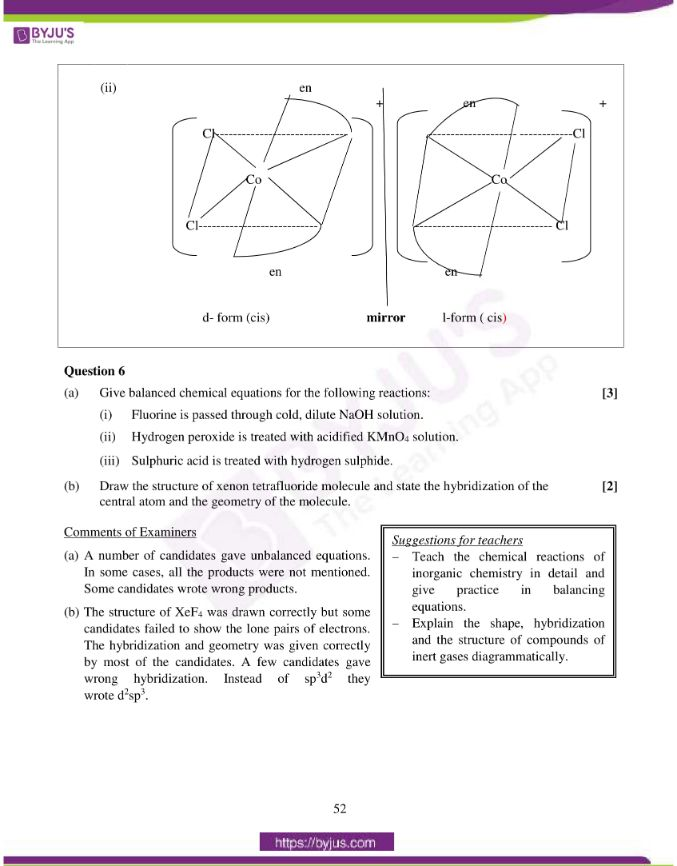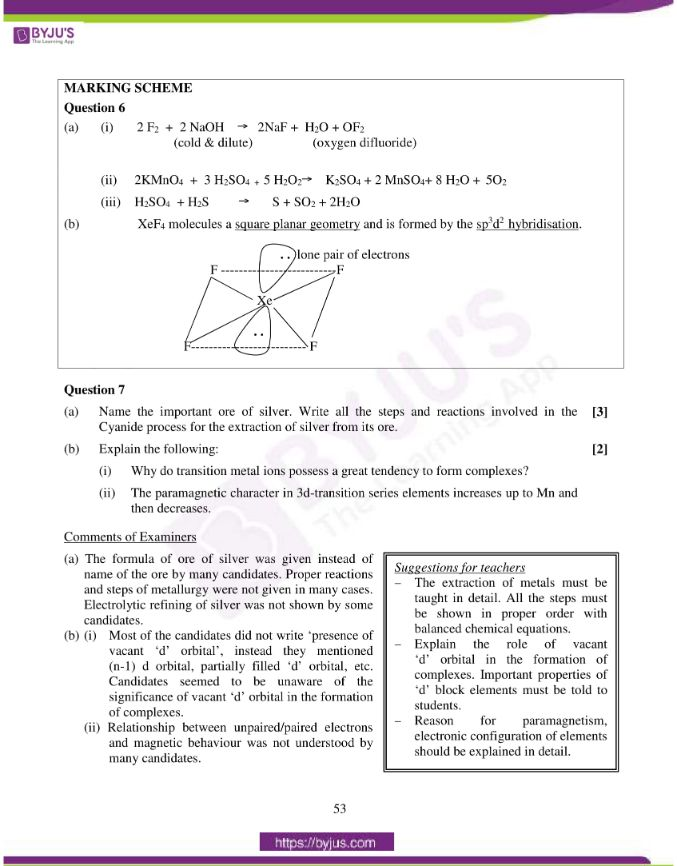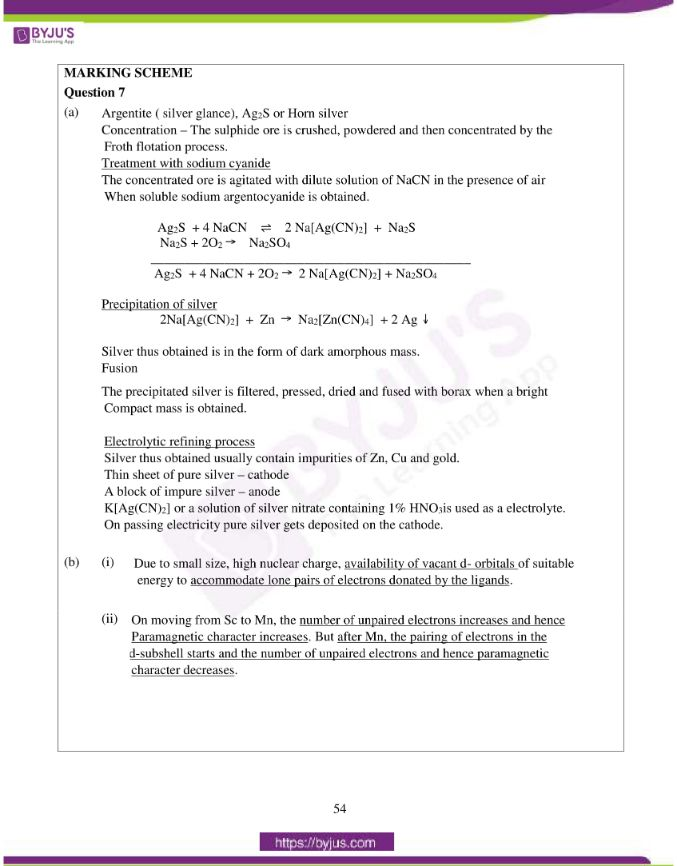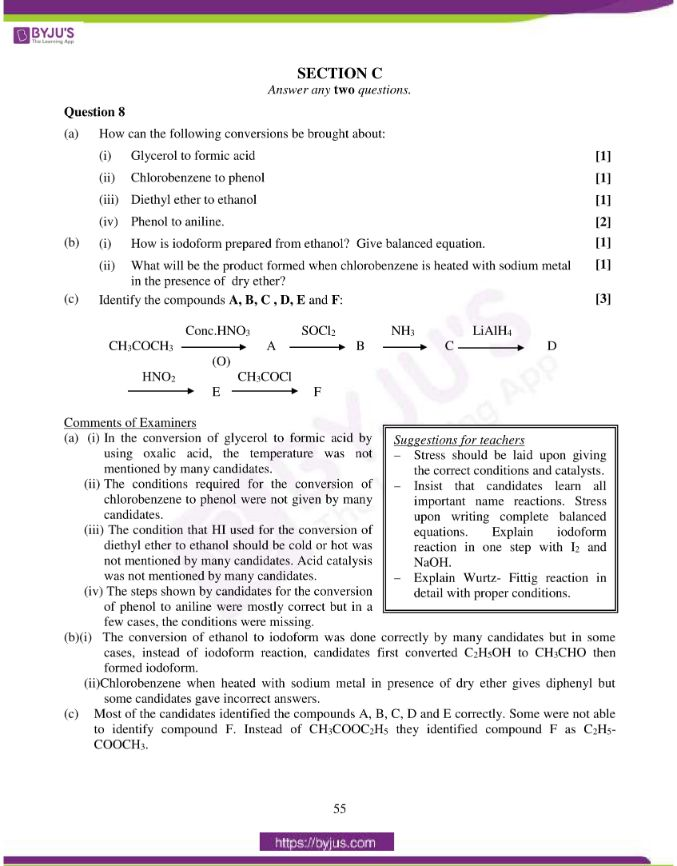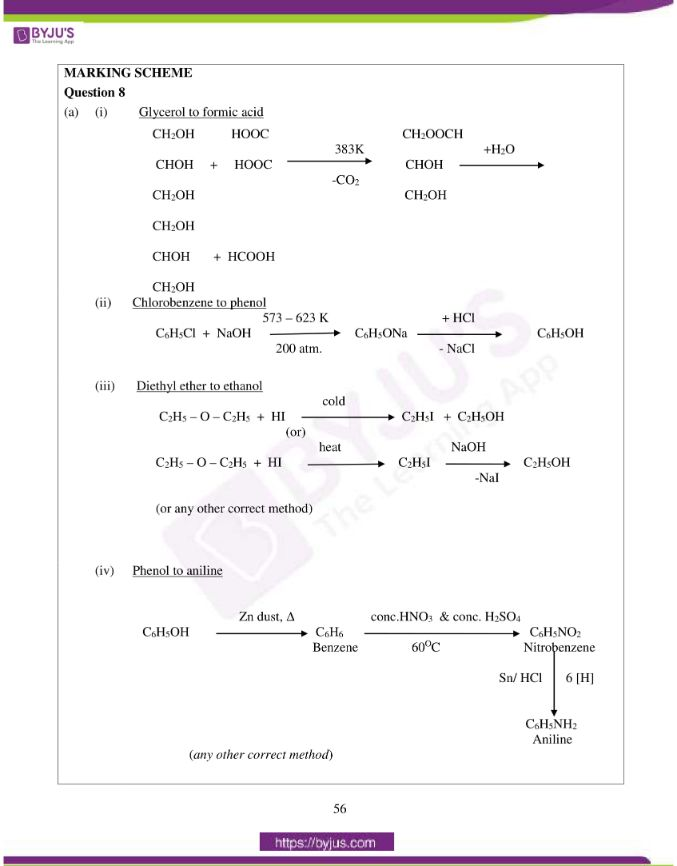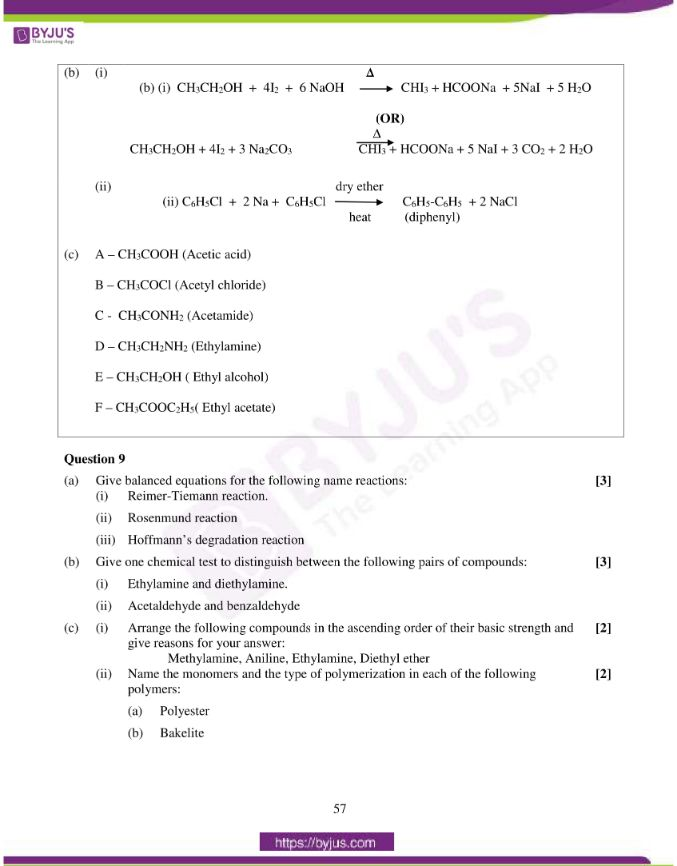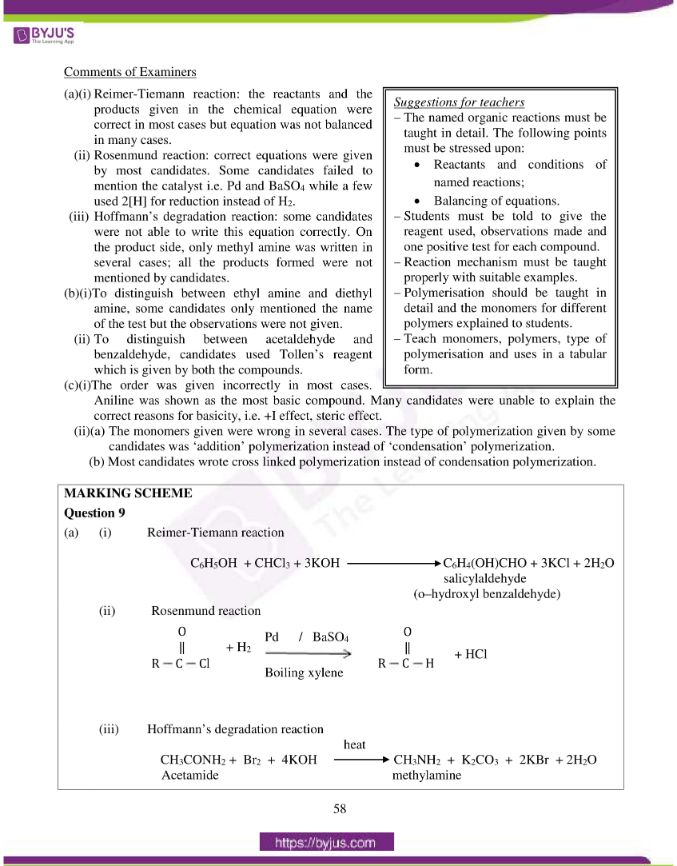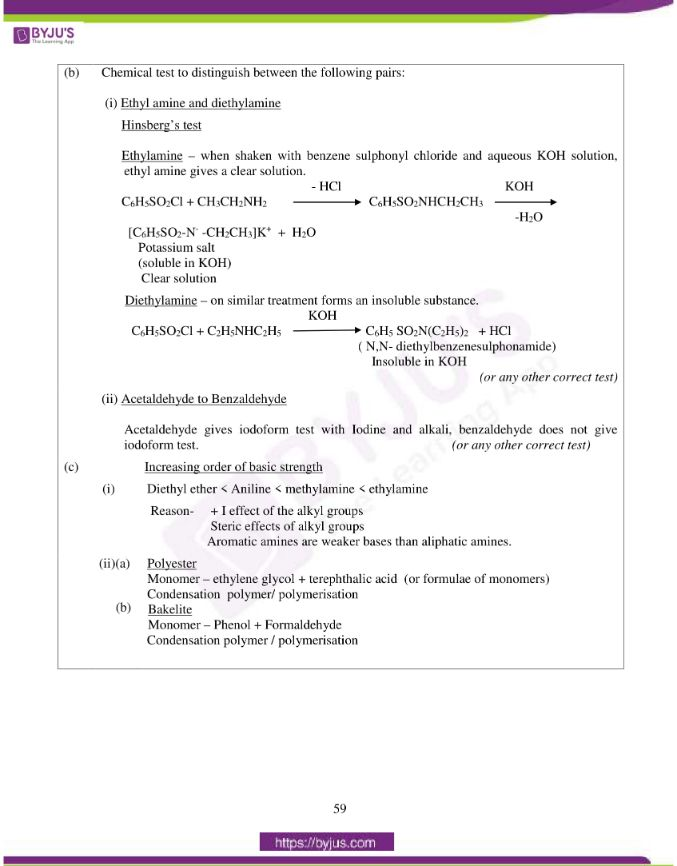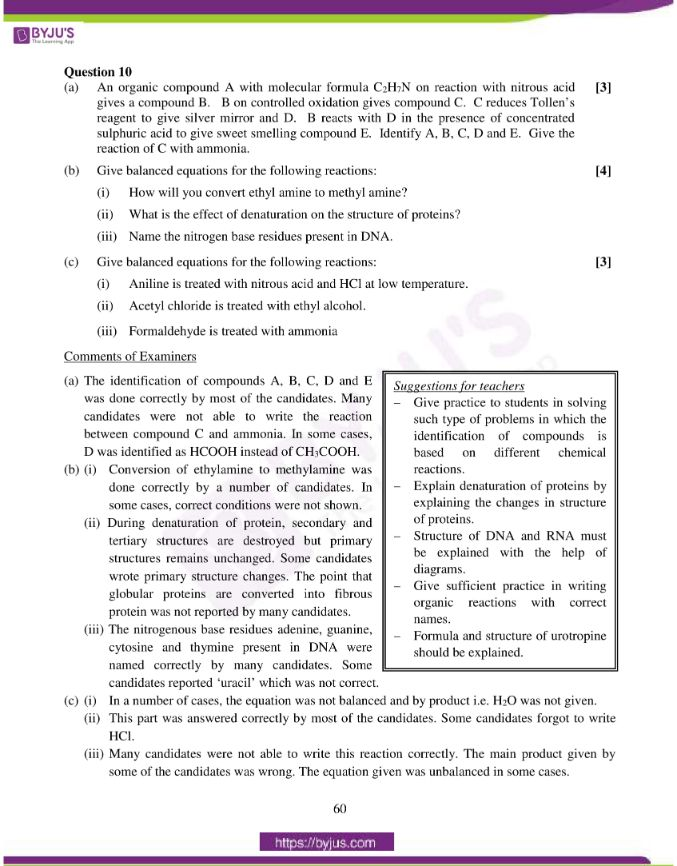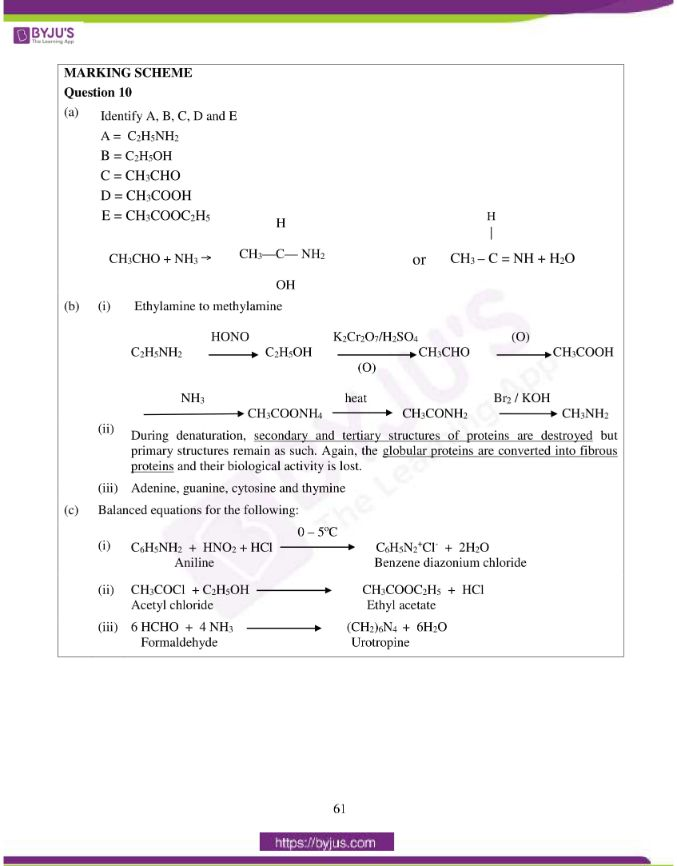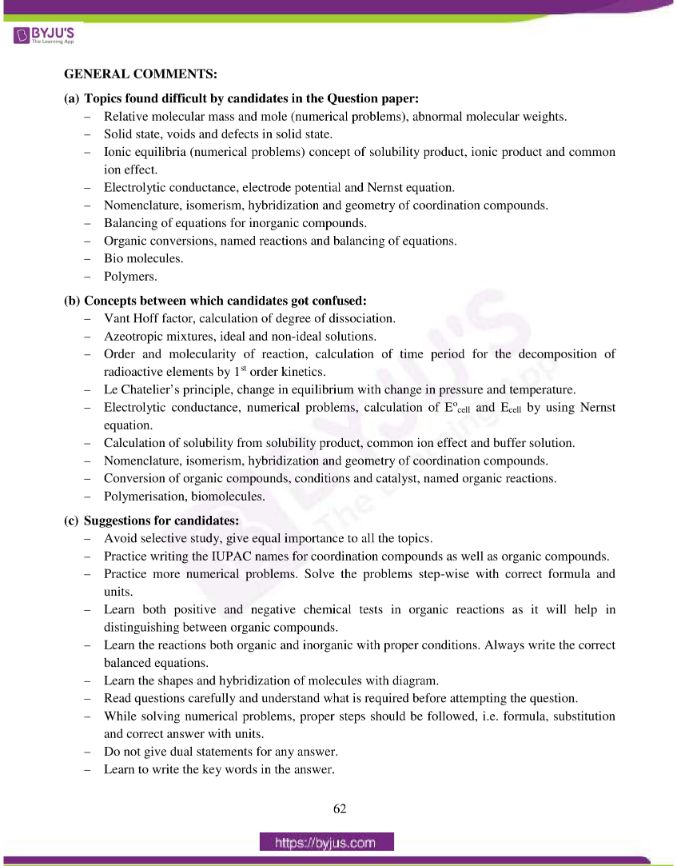### Difficult Topics of ISC Class 12 Chemistry Paper 2015

Topics which students found difficult while solving the Chemistry 2015 paper are mentioned below:

• Relative molecular mass and mole (numerical problems), abnormal molecular weights.
• Solid state, voids and defects in solid state.
• Ionic equilibria (numerical problems) concept of solubility product, ionic product and common ion effect.
• Electrolytic conductance, electrode potential and Nernst equation.
• Nomenclature, isomerism, hybridization and geometry of coordination compounds.
• Balancing of equations for inorganic compounds.
• Organic conversions, named reactions and balancing of equations.
• Biomolecules.
• Polymers.

### Confusing ISC Class 12 Chemistry Questions 2015

Chemistry concepts between which students got confused during the exam are mentioned below.

• Vant Hoff factor, calculation of degree of dissociation.
• Azeotropic mixtures, ideal and non-ideal solutions.
• Order and molecularity of reaction, calculation of time period for the decomposition of radioactive elements by 1st order kinetics.
• Le Chatelier’s principle, change in equilibrium with change in pressure and temperature.
• Electrolytic conductance, numerical problems, calculation of Eocell and Ecell by using Nernst equation.
• Calculation of solubility from solubility product, common ion effect and buffer solution.
• Nomenclature, isomerism, hybridization and geometry of coordination compounds.
• Conversion of organic compounds, conditions and catalysts, named organic reactions.
• Polymerisation, biomolecules.

## ISC Class 12 Chemistry Question Paper Solution 2015

### Question 1:

(a) Fill in the blanks by choosing the appropriate word/words from those given in the

brackets:

(increases, decreases, positive, efficient, 68, non-efficient, no α-hydrogen, α-hydrogen, negative, Rosenmund’s, greater, Cannizzaro, 74, common-ion effect, lesser, buffer action, diamagnetic, paramagnetic)

(i) The more__________ the standard reduction potential of a metal, the ________ is its ability to displace hydrogen from acids.

(ii) Both ccp and hcp are ____________ packings and occupy about _________% of the available space.

(iii) Solubility of silver chloride _____________ in the presence of sodium chloride because of _____________.

(iv) Benzaldehyde undergoes ___________ reaction on treatment with concentrated sodium hydroxide because it has ___________ atom.

(v) The transition metals show ______________ character because of the presence of unpaired electrons and Cu+ is _____________because its electronic configuration

is [Ar]3d10.

(b) Complete the following statements by selecting the correct alternative from the choices given:

(i) The molal freezing point constant of water is 1·86 K kg mol-1. Therefore, the freezing point of 0·1M NaCl solution in water is expected to be:

1. -1·86oC
2. -0·372oC
3. -0·186oC
4. +0·372oC

(ii) For a first order reaction the rate constant for decomposition of N2O5 is 6×10-4sec-1. The half-life period for the decomposition in seconds is:

1. 11·55
2. 115·5
3. 1155
4. 1·155

(iii) When acetaldehyde is treated with Grignard reagent, followed by hydrolysis the product formed is:

1. Primary alcohol
2. Secondary alcohol
3. Carboxylic acid
4. Tertiary alcohol

(iv) The geometry of XeF6 molecule and the hybridization of Xe atom in the molecule is:

1. Distorted octahedral and sp3d3
2. Square planar and sp3d2
3. Pyramidal and sp3
4. Octahedral and sp3d3

(v) In the complexes [Fe(CN)6]3- and [Pt(en) (H2O)2(NO2)(Cl)]2+ the respective oxidation numbers of central metal atoms are :

1. + 3 and +4
2. +6 and +4
3. +6 and +3
4. +3 and +3

(i) What is the effect of temperature on the ionic product of water? How will it change the pH value of a neutral solution?

(ii) How many hours does it take to reduce 3 moles of Fe3+ to Fe2+ with 2·0 A current intensity?

(iii) How is urea prepared by Wohler synthesis?

(iv) Two liquids A and B form type II non ideal solution which shows a minimum in its temperature -mole fraction plot (T-χ diagram). Can the two liquids be completely separated by fractional distillation?

(v) The aqueous solution of sodium acetate is basic. Explain.

(d) Match the following:

 (i) Disaccharide (a) Lucas reagent (ii) Carbylamine (b) Condensation polymer (iii) Dacron (c) Obnoxious smell (iv) Low spin complex, d2sp3 (d) Sucrose (v) Anhydrous ZnCl2 + conc. HCl (e) Hexaamminecobalt(III)ion

(ii) efficient, 74

(iii) decreases, common-ion effect

(iv) Cannizzaro, no α- hydrogen

(v) paramagnetic, diamagnetic

(b) (i) (2) -0·372oC

(ii) (3) 1155

(iii) (2) secondary alcohol

(iv) (1) distorted octahedral and sp3d3

(v) (1) +3 and +4

(c) (i) Ionic product increases with increase in temperature because the dissociation of water

increases with increase of temperature. With increase in concentration of H3O+ ions, pH of the neutral solution will decrease.

(ii) Reduction of 1mol of Fe3+ requires = 96500 C

Reduction of 3 mol of Fe3+ require = 3 x 96500 C = 2.895 X 105C

Q = I x t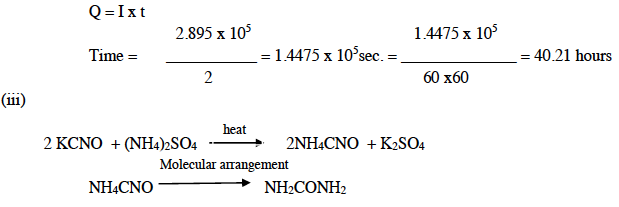(iv) The two liquids cannot be separated completely by fractional distillation because they form a constant boiling azeotropic mixture, therefore at a definite composition both the liquids will distil over without any change in composition.

(v) Sodium acetate undergoes anionic hydrolysis and forms weakly dissociated CH3COOH and highly dissociated NaOH.

(d)

 (i) Disaccharide (d) Sucrose (ii) Carbylamine (c) Obnoxious smell (iii) Dacron (b) Condensation polymer (iv) Low spin complex, d2sp3 (e) Hexaamminecobalt(III)ion (v) Anhydrous ZnCl2 + conc. HCl (a) Lucas reagent

### Question 2:

(a) (i) A solution containing 0·5 g of KCl dissolves in 100 g of water and freezes at – 0·24oC. Calculate the degree of dissociation of the salt. (Kf for water = 1.86oC)

Atomic weights [K = 39, Cl = 35·5]

(ii) If 1·71 g of sugar (molar mass = 342) are dissolved in 500 ml of an aqueous solution at 300 K, what will be its osmotic pressure?

(iii) 0·70g of an organic compound when dissolved in 32g of acetone produces an elevation of 0·25oC in the boiling point. Calculate the molecular mass of organic compound (Kb for acetone = 1·72 K kg mol-1).

(b) (i) What is the difference between order of a reaction and the molecularity of a

reaction?

(ii) A substance decomposes by following first order kinetics. If 50% of the compound is decomposed in 120 minutes, how long will it take for 90% of the compound to decompose?

(c) Name the crystal structure of the copper metal.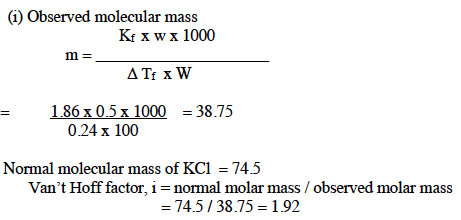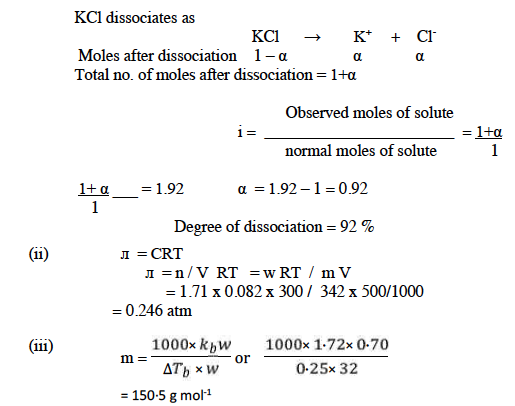(b) (i) Difference between order of reaction and molecularity of a reaction:

 Order of reaction Molecularity of reaction It is equal to the sum of the powers of the molar concentrations of the reactants in the rate law. It is equal to the total number of molecules of the reactants which take part in a single step chemical reaction. It may be in fractions or may be zero or negative. It is always a positive whole number value. It is for the overall reaction and an experimentally determined quantity. It is a theoretical concept and depends on the rate determining step in the reaction mechanism because overall molecularity of a complex reaction has no significance.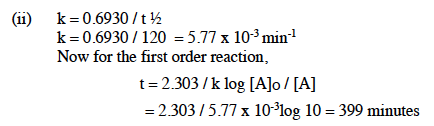(c) Face centered cubic (fcc) or Cubic close packing (ccp)

### Question 3:

(a) (i) Chromium metal crystallises with a body centered cubic lattice. The edge length of the unit cell is found to be 287 pm. Calculate the atomic radius. What would be the density of chromium in g / cm3? (atomic mass of Cr = 52·99)

(ii) Why does sodium chloride on heating with sodium vapours acquire a yellow colour?

(iii) The equilibrium constant for the reaction:

N2(g) + 3H2(g) ⇌ 2NH3(g) at 715 K, is 6.0×10-2.

If, in a particular reaction, there are 0·25 mol L-1 of H2 and 0·06 mol L-1 of NH3 present, calculate the concentration of N2 at equilibrium.

(iv) Calculate the concentration of OH ions in solution when [H+] = 6.2 x 10-2 molL-1.

(v) State the Le-Chatelier’s principle.

(b) For a crystal of sodium chloride, state:

(i) The type of lattice in which it crystallises.

(ii) The coordination number of each sodium ion and chloride ion in the crystal lattice.

(iii) The number of sodium ions and chloride ions present in a unit cell of sodium chloride.

(iv) The structural arrangement of the sodium chloride crystal.

(c) Consider the following reaction:

N2O4(g) + Heat ⇌ 2NO2(g)

How is the composition of equilibrium mixture affected by:

(i) a change in temperature

(ii) a change in pressure

(iii) a change in concentration of N2O4

(iv) the removal of NO2 from the reaction mixture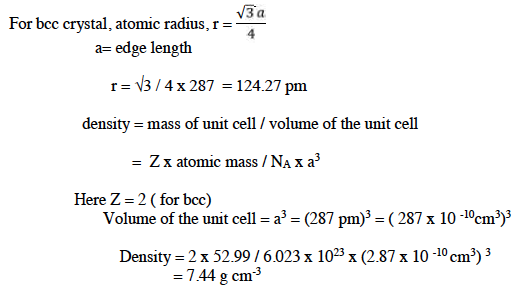(ii) On heating sodium chloride with sodium vapours, the chloride ions diffuse to the surface of the crystals and combine with Na atoms which get ionized to Na+ ions by losing electrons. These electrons get trapped in anion vacancies and act as F- centres which impart colour to the crystal.

(iii) For the reaction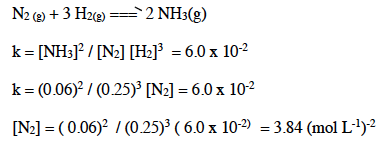(iv) KW = [H+] [OH] [OH] = KW / [H+] = 10-14 / 6.2 x 10-2 = 1.6 x 10 -11mol L-1

(v) If an equilibrium is subjected to a stress (change in concentration, pressure or temperature etc.) equilibrium shifts in such a way so as to undo or decrease the effect of stress imposed.

(b) (i) Face-centered cubic lattice (fcc) (or) cubic close-packing(ccp)

(ii) Coordination number of each Na+ ion as well as Cl ion is 6.

(iii) The unit cell of sodium chloride possess 4 sodium ions and 4 chloride ions.

(iv) Octahedral.

(c) (i) Increase in temperature favors the forward reaction, concentration of NO2 increases and vice versa.

(ii) Increase in pressure favors backward reaction and vice versa.

(iii) Addition of N2O4 favors the forward reaction and vice versa.

(iv) Removal of NO2 increases the rate of forward reaction or equilibrium will shift to the forward direction.

### Question 4:

(a) The specific conductance of a 0.01 M solution of acetic acid at 298 K is 1.65 x 10-4 ohm-1 cm-1.The molar conductance at infinite dilution for H+ ion and CH3COO ion are 349.1 ohm-1 cm2mol-1 and 40.9 ohm-1cm2mol-1 respectively.

Calculate:

(i) Molar conductance of the solution.

(ii) Degree of dissociation of CH3COOH.

(iii) Dissociation constant for acetic acid.

(b) (i) Calculate the e.m.f. of the following cell reaction at 298 K:

Mg(s) + Cu2+ (0.0001 M) → Mg2+ (0.001M) + Cu(s)

The standard potential (E0) of the cell is 2.71 V.

(ii) The solubility product (Ksp) of BaSO4 is 1.5 x 10-9.Calculate the solubility of barium sulphate in pure water and 0.1 M BaCl2.

(c) Explain the following :

(i) When NH4Cl and NH4OH are added to a solution containing both, Fe3+ and Ca2+ ions, which ion is precipitated first and why?

(ii) Dissociation of H2S is suppressed in acidic medium.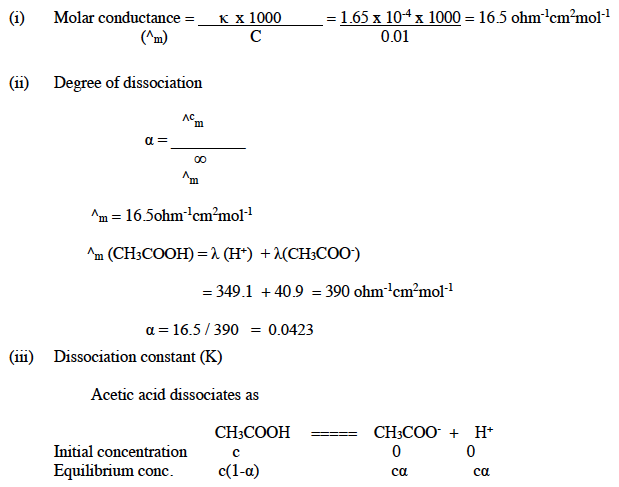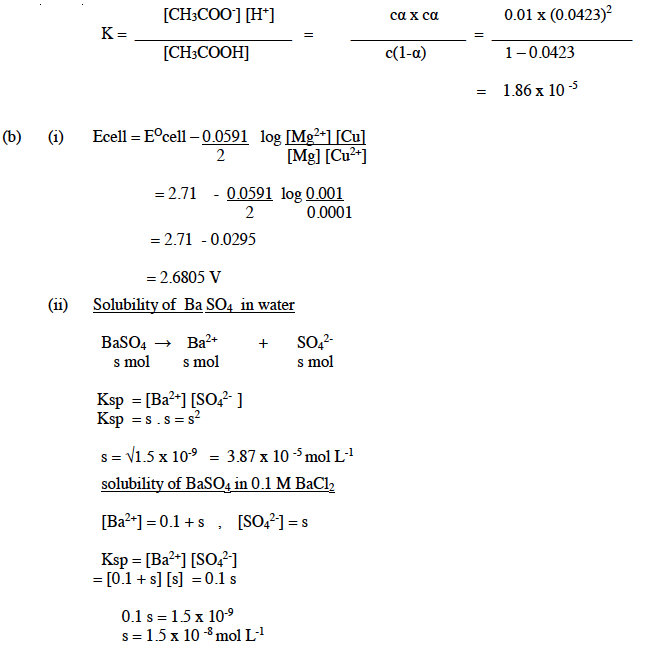(c) (i) Fe3+ ion will precipitate out first.

NH4OH ⇌ NH4+ + OH

NH4Cl ⇌ NH4+ Cl (eq. or common ion effect)

Due to the common ion effect less OH ions are produced, which are large enough to cause the precipitation of Fe3+ ions. As its solubility product is less (Ksp is less for Fe(OH)3 and

Ksp is more for Ca(OH)2).

(ii) This is due to the common ion effect. The suppression of degree of dissociation of a weak electrolyte by (H2S) the addition of a strong electrolyte (HCl) having a common ion with the weak electrolyte (H2S).

### Question 5:

(a) Write the IUPAC names of the following coordination compounds:

(i) [Cr(NH3)4(H2O)2]Cl3

(ii) [PtCl2(NH3)4] [PtCl4]

(b) State the hybridization and magnetic property of [Fe(CN)6]3- ion according to the valence bond theory.

(c) (i) What type of isomers are [Co(NH3)5Br]SO4 and [Co(NH3)5SO4]Br.? Give a chemical test to distinguish between them.

(ii) Write the structures of optical isomers of the complex ion [Co(en)2Cl2]+

(ii) tetraamminedichloroplatinum(IV)tetrachloroplatinate(II)

(b) d2sp3 hybridisation and paramagnetic

(c) (i) Ionisation isomers

One of these is red-violet and forms a precipitate with BaCl2 indicating that sulphate ion is outside the coordination sphere. The second one is red and does not form ppt. with BaCl2 but forms a ppt. of AgBr with AgNO3 indicating that bromide ion is outside the coordination sphere.

(ii)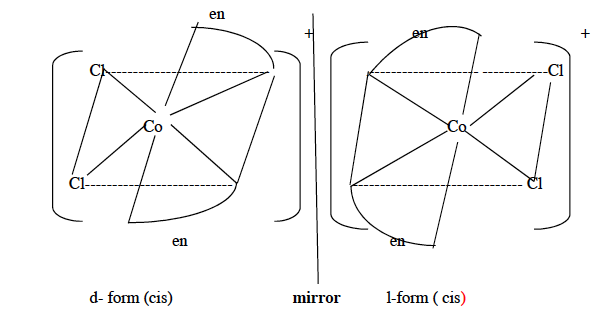### Question 6:

(a) Give balanced chemical equations for the following reactions:

(i) Fluorine is passed through cold, dilute NaOH solution.

(ii) Hydrogen peroxide is treated with acidified KMnO4 solution.

(iii) Sulphuric acid is treated with hydrogen sulphide.

(b) Draw the structure of xenon tetrafluoride molecule and state the hybridization of the central atom and the geometry of the molecule.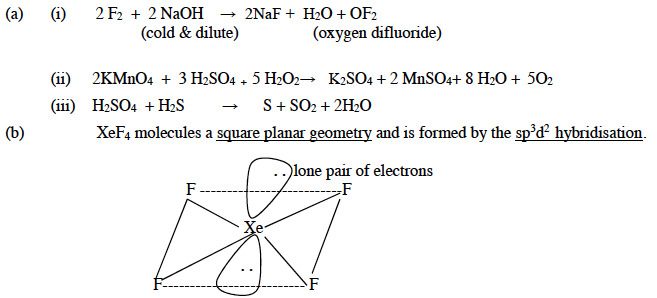### Question 7:

(a) Name the important ore of silver. Write all the steps and reactions involved in the Cyanide process for the extraction of silver from its ore.

(b) Explain the following:

(i) Why do transition metal ions possess a great tendency to form complexes?

(ii) The paramagnetic character in 3d-transition series elements increases up to Mn and then decreases.

Answer: (a) Argentite ( silver glance), Ag2S or Horn silver

Concentration – The sulphide ore is crushed, powdered and then concentrated by the Froth flotation process.

Treatment with sodium cyanide

The concentrated ore is agitated with dilute solution of NaCN in the presence of air

When soluble sodium argentocyanide is obtained.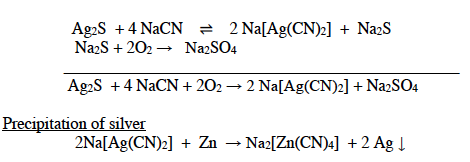Silver thus obtained is in the form of dark amorphous mass.

Fusion

The precipitated silver is filtered, pressed, dried and fused with borax when a bright

Compact mass is obtained.

Electrolytic refining process

Silver thus obtained usually contains impurities of Zn, Cu and gold.

Thin sheet of pure silver – cathode

A block of impure silver – anode

K[Ag(CN)2] or a solution of silver nitrate containing 1% HNO3 is used as an electrolyte.

On passing electricity pure silver gets deposited on the cathode.

(b) (i) Due to small size, high nuclear charge, availability of vacant d- orbitals of suitable energy to accommodate lone pairs of electrons donated by the ligands.

(ii) On moving from Sc to Mn, the number of unpaired electrons increases and hence Paramagnetic character increases. But after Mn, the pairing of electrons in the d-subshell starts and the number of unpaired electrons and hence paramagnetic character decreases.

### Question 8:

(a) How can the following conversions be brought about:

(i) Glycerol to formic acid

(ii) Chlorobenzene to phenol

(iii) Diethyl ether to ethanol

(iv) Phenol to aniline.

(b) (i) How is iodoform prepared from ethanol? Give a balanced equation.

(ii) What will be the product formed when chlorobenzene is heated with sodium metal in the presence of dry ether?

(c) Identify the compounds A, B, C , D, E and F: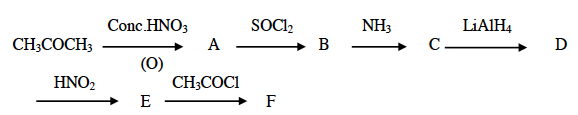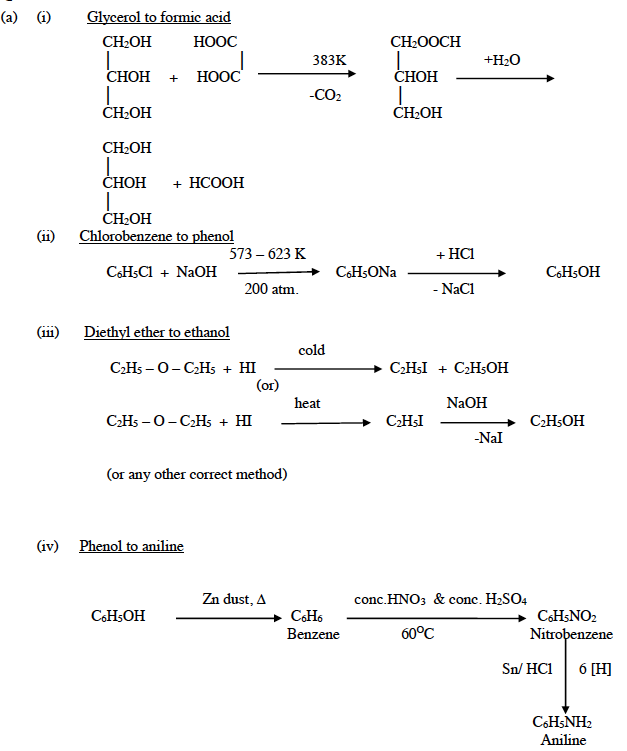(b)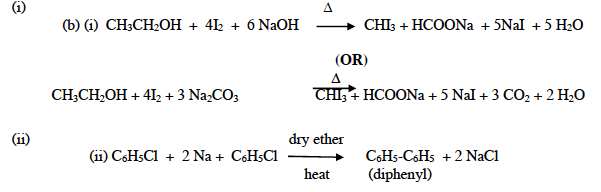(c) A – CH3COOH (Acetic acid)

B – CH3COCl (Acetyl chloride)

C – CH3CONH2 (Acetamide)

D – CH3CH2NH2 (Ethylamine)

E – CH3CH2OH ( Ethyl alcohol)

F – CH3COOC2H5( Ethyl acetate)

### Question 9:

(a) Give balanced equations for the following name reactions:

(i) Reimer-Tiemann reaction.

(ii) Rosenmund reaction

(b) Give one chemical test to distinguish between the following pairs of compounds:

(i) Ethylamine and diethylamine.

(ii) Acetaldehyde and benzaldehyde

(c) (i) Arrange the following compounds in the ascending order of their basic strength and give reasons for your answer:

Methylamine, Aniline, Ethylamine, Diethyl ether

(ii) Name the monomers and the type of polymerization in each of the following polymers:

(a) Polyester

(b) Bakelite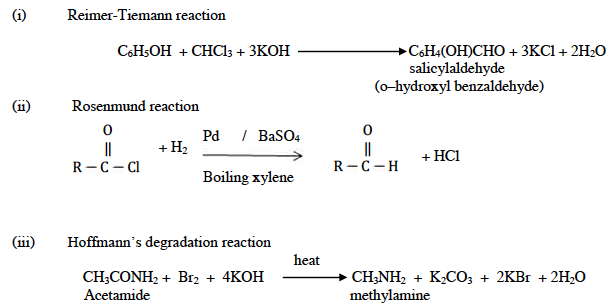(b) Chemical test to distinguish between the following pairs:

(i) Ethyl amine and diethylamine

Hinsberg’s test

Ethylamine – when shaken with benzene sulphonyl chloride and aqueous KOH solution, ethyl amine gives a clear solution.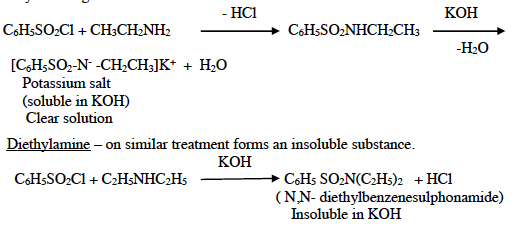(ii) Acetaldehyde to Benzaldehyde

Acetaldehyde gives iodoform test with Iodine and alkali, benzaldehyde does not give

iodoform test.

(c) (i) Increasing order of basic strength

Diethyl ether < Aniline < methylamine < ethylamine

Reason:

• +I effect of the alkyl groups
• Steric effects of alkyl groups
• Aromatic amines are weaker bases than aliphatic amines.

(ii)(a) Polyester

• Monomer – ethylene glycol + terephthalic acid (or formulae of monomers)
• Condensation polymer/ polymerisation

(b) Bakelite

• Monomer – Phenol + Formaldehyde
• Condensation polymer / polymerisation

### Question 10:

(a) An organic compound A with molecular formula C2H7N on reaction with nitrous acid gives a compound B. B on controlled oxidation gives compound C. C reduces Tollen’s reagent to give silver mirror and D. B reacts with D in the presence of concentrated sulphuric acid to give sweet smelling compound E. Identify A, B, C, D and E. Give the reaction of C with ammonia.

(b) Give balanced equations for the following reactions:

(i) How will you convert ethyl amine to methyl amine?

(ii) What is the effect of denaturation on the structure of proteins?

(iii) Name the nitrogen base residues present in DNA.

(c) Give balanced equations for the following reactions:

(i) Aniline is treated with nitrous acid and HCl at low temperature.

(ii) Acetyl chloride is treated with ethyl alcohol.

(iii) Formaldehyde is treated with ammonia

Answer: (a) Identify A, B, C, D and E

A = C2H5NH2

B = C2H5OH

C = CH3CHO

D = CH3COOH

E = CH3COOC2H5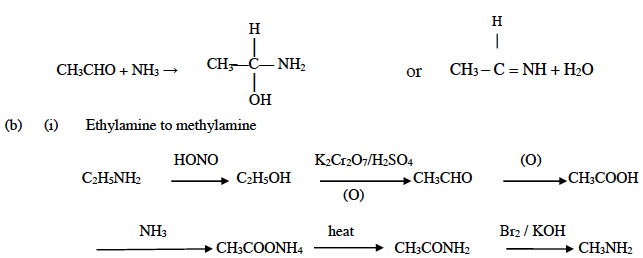(ii) During denaturation, secondary and tertiary structures of proteins are destroyed but primary structures remain as such. Again, the globular proteins are converted into fibrous proteins and their biological activity is lost.

(iii) Adenine, guanine, cytosine and thymine

(c) Balanced equations for the following: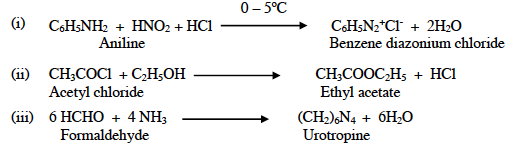ISC Class 12 Chemistry Question Paper Solution 2015 must have helped students in analysing their current level of exam preparation. Keep practising more questions and stay tuned to BYJU’S for the latest update on ICSE/CBSE/State Boards/Competitive exams. Also, download the BYJU’S App to get interactive study related videos.